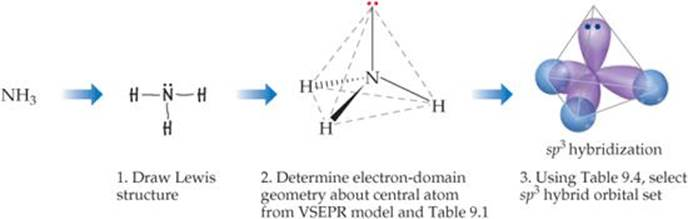# How do I determine the hybridization and bonding in ammonia?

Jun 22, 2017

Here's how to do it.

#### Explanation:

You draw the Lewis structure (Step 1) and use VSEPR theory to determine its electron domain geometry as tetrahedral and its molecular shape (Step 2) as a trigonal pyramid.Then you consult a table like the one below.You find that the $\text{N}$ atom uses ${\text{sp}}^{3}$ orbitals, so you draw four tear-drop shapes pointing towards the corners of a tetrahedron (Step 3).

The $\text{H}$ atoms use their $\text{1s}$ orbitals for bonding, so you draw small circles intersecting with three of the $\text{sp}$3 orbitals.

That shows that the $\text{N-H}$ bonds are σ bonds formed by the overlap of ${\text{sp}}^{3}$ orbitals of $\text{N}$ and $\text{1s}$ orbitals of $\text{H}$.

However, the molecular orbitals include both the $\text{N}$ and $\text{H}$ nuclei.In the diagram above, the bond-line drawing shows that the $\text{H}$ nuclei are completely inside the larger teardrop-shaped $\text{sp"^3"-s}$ σ molecular orbitals.